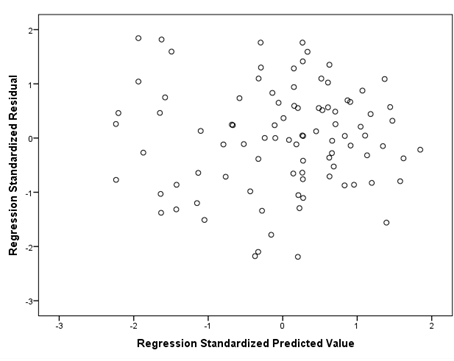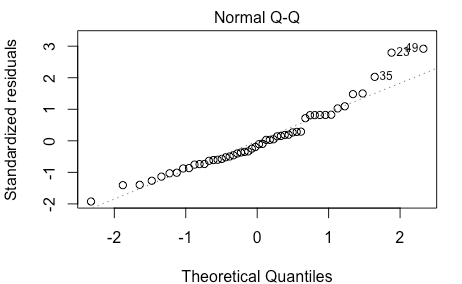Quick access through the institutional single sign-on
Skip this for now
|
Public Access HereLogging You In!
Skip this for now
|
Man Met Access Heremenu

# Parametric Assumptions Quiz

Part 1: Interpreting Q-Q plots

1. Are the graphs below homoscedastic or not?2. Do the graphs below pass the normality parametric assumption and why?3. Is the graph below linear and why?

4. Complete the table: Read the examples below and state if they will have a positive, negative or no correlation: